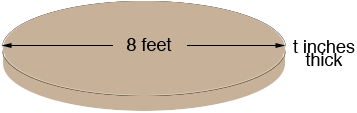SEARCH HOMEMath Central Quandaries & QueriesHi, I have a tank that needs a base of cement put in it. I don't know how to do this. The tank is 8 feet across and I want the cement to be 5 or 6 inches deep. Can you please tell me how much cement I need to do this. KennHi Kenn,

I drew a diagram of what you described.The volume of a circular disk is π r2 t where r is the radius ant t is the thickness. Since this is concrete I expect you want the volume in cubic yards so you should first convert the measurements to yards. There are 3 feet in a yard so the radius is 8/3 yards and there are 36 inches in a yard so the thickness is t/36 yards. Thus the volume is

π r2 t = π (4/3) 2 × ( t/36) = 0.16 t cubic yards where t is in inches.

Hence if the base is 5 inches thick the volume is 0.16 ×5 = 0.78 cubic yards.

HarleyMath Central is supported by the University of Regina and The Pacific Institute for the Mathematical Sciences.